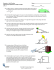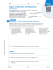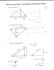# Trig Applications ws day 2 geo 14

## Transcription

Trig Applications ws day 2 geo 14
```Geometry
Trig Applications Worksheet Day 2
Name____________________________________
Solve the following problems. (All answers to 2 decimals places, unless otherwise instructed.)
1) A tree casts a shadow 21 m long. The angle of elevation of the sun is
55. What is the height of the tree?
2) You are flying a kite and have let out 30 ft of string but it got caught in
a 8 ft tree. What is the angle of elevation to the location of the kite?
3) A guy wire reaches from the top of a 120 m television transmitter
tower to the ground. The wire makes a 68 angle with the ground. Find
the length of the guy wire.
4) An airplane climbs at an angle of 16 with the ground. Find the ground
distance the plane travels as it moves 2500 m through the air.
5) A lighthouse operator sights a sailboat at an angle of depression of 12.
If the sailboat is 80 m away, how tall is the lighthouse?
6. a) How long is the guy wire?
b) What is the angle formed
between the guy wire and the
ground?
7. a) A ramp is 18 m long. If the
horizontal distance of the ramp is
17m, what is the vertical distance?
b) What is the angle of elevation of
the ramp?
8. Sharon is flying a kite on a string 130 m long. Determine the height of the kite if the string is at an angle
of 37 to the ground.
9. An airplane is flying at an altitude of 6000 m over the ocean directly
toward an island. When the angle of depression of the coastline from the
airplane is 14, how much farther does the airplane have to fly before it
crosses the coast?
10. A loading ramp is 25 m long with a height of 10 m. What is the
horizontal distance of the ramp and what is the angle of incline that the
ramp forms with the ground?
11. A telephone pole casts a shadow 18 m long when the sun’s rays strike
the ground at an angle of 70. How tall is the pole?
12. Mike looks out the attic window of his home, which is 22 ft above the
ground. At an angle of elevation of 35 he sees a bird sitting at the very top
of the large high rise apartment building down the street. How tall is the
high rise apartment building, if the two buildings are 75 ft apart?
13. Using the drawbridge diagram, determine the distance from one side to the other. (exact answer)
45°
40 ft
45°
40 ft
14. Now that you know the distance from side to side, determine how high the drawbridge would be if the
angle of elevation was 60.(exact answer)
60°
60°
40 ft
15. How far apart would the drawbridge be if the angle of elevation of the drawbridge was 20?
x
20°
20°
```

### Angle of Elevation and Depression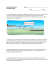### installation of an angle board * * * thermopian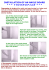### File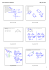### AC Geo/Adv.Alg Unit 1 Worksheet 11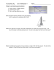### Worksheet - Multi-Step Trigonometry Problem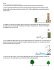### MCR3U - 4.2 - Applications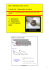### G.SRT.8 Wkst 2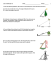### If ABC is obtuse, what are the restrictions on x? ∠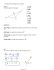### File - HHS Pre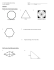### ENS1101 - Engineering Mechanics Assignment Lecturer: Dr Shuo LI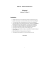### Inverse Trig Functions You know how to find the missing side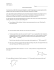### Document 6592969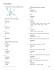### Build an Angle Setting Jig to Sharpening Plane Irons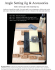### Reflection (from Mulan) Rose is Rose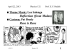### Wkst- Law of Sines-Area of Triangle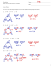### Adjustable Angle Composite Sheeter Options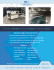### Chapter 6 THE D HULK AKA RULK! Review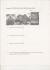### trigonometry applications in right triangles - pdf